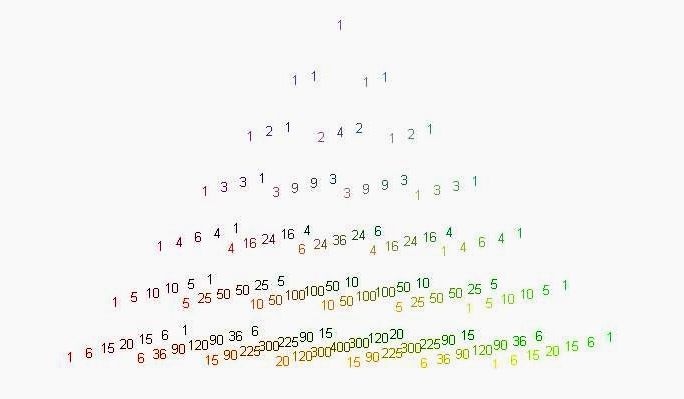## Pascal’s Pyramid (part 1 of 3)

byIn an earlier post, Ξ had mentioned a question from Who Wants To Be A Millionaire that made reference to “a famous “pyramid” of numbers that starts with the number one on top”.  The intended answer was “Pascal’s Triangle” (my emphasis).

That got me wondering what a three dimensional analogue of the Pascal Triangle might look like.

Today we’ll explore a Pascal-like construction of a square pyramid.   The top-most point on the pyramid will be assigned the value 1.  As we move down the pyramid,   let’s assign each point a value  by taking the sum of the 4 terms directly above and adjacent to each point.

If you think about the 4 outer faces, each point along a face only has two points that lie above it.  Thus if we restrict our attention just to one outer face, our construction is identical to the two dimensional Pascal triangle, and for  each face we should get the familiar binomial coefficients.

One can describe  how a given entry depends on those above it:  If we let f(n, a, b) be the entry in the nth layer down from the top, in row a and column b, then the entries that lie above will be in layer n-1, their row will be a or a-1, and their column will be b or b-1.  Thus

f (n, a, b) = f(n-1, a-1,b-1) + f(n-1, a, b-1) + f(n-1, a-1, b) + f(n-1, a, b)

with f(0,0,0)=1, and f understood to be zero if any of the parameters get out of range (e.g. if a or b > n, or is negative).

Numerically, we get the following pattern (for 0 ≤ n ≤ 6):For example, the entries in the fifth layer are:Each entry appears to be the product of the first entry in its row, with the first entry in its column.  Since we know the row and column values are binomial coefficients, we conjecture that$f(n,a,b) = {n \choose a} {n \choose b}$.

To prove this, we start by verifying that when n=0, the product of the binomial coefficients is equal to 1.  Proceeding inductively, we assume that the result holds for n-1, and we compute f(n,a,b):${ {n-1} \choose {a-1}} {{n-1} \choose {b-1}} + {{n-1} \choose {a}} { {n-1} \choose {b-1}} + {{n-1} \choose {a-1}}{{n-1} \choose {b}} + {{n-1} \choose {a}} {{n-1} \choose {b}}$$= \bigg( {{n-1} \choose {a-1} } + {{n-1} \choose {a}} \bigg) \bigg( {{n-1} \choose {b-1}} + {{n-1} \choose {b}} \bigg)$$= { {n}\choose {a}} {{n} \choose {b}}$

QED by induction; each entry in Pascal’s Square Pyramid is a product of two binomial coefficients, and is equal to the sum of the four terms immediately above it.

Coming upTetrahedral pyramids (2/3);  Sierpinski’s Pascal Pyramid (3/3)

### 7 Responses to “Pascal’s Pyramid (part 1 of 3)”

1.David Petersen Says:

Awesome! I love this as a “mathematical induction” problem. The motivation beginning with the gameshow, a cool visual, a pattern that is not too hard to discern, and a review of combinatorics.

2.David Petersen Says:

Oh yeah, and some nice factoring in a non-standard presentation.

(Not sure why I put quotations in the previous post.)

3.Tetrahedral Pyramids (part 2 of 3) « 360 Says:

[…] Pyramids (part 2 of 3) By TwoPi The first post in this series, Pascal’s Pyramid (part 1 of 3), explored number patterns that arise in a 3 dimensional version of Pascal’s Triangle: a […]

4. Mathematics Carnival 51 - squareCircleZ Says:

[…] offers us Pascal’s Pyramid (part 1 of 3), where he ponders the nature of a three dimensional analogue of the Pascal Triangle. Blog home: […]

5.jd2718 Says:

OK. This is definitely cool.

6.Jose Says:

im sorry i didn’t took the time to read the other posts, perhaps i might repeat some comments, yet id like to share my new art thing. i just constructed a pascale’s pyramid, but the one which contains the coefficients for trinomial expansions (rows 0 until 4, i was too impatient to move onto the next rows). The pyramid had a triangular base instead of squared one, as shown in the article (could that be the 4″nomial” expansion coeffs?).
I took a few pictures to share with anybody who could be interested, so if you wish, I could email you these. Cheers

7.Max Swatek Says:

I my self am a tenth grade high school student who is most happy to find other people experimenting with higher order Pascal relations. Last year when i was in grade 9 i got really bored in class and started working with the Pascal triangle but it didn’t take long for me to get bored with that and start working on a Pascal pyramid, i noticed many interesting patterns that occurred both in the 3D pyramid and in the digitalized form of the pyramid, but all that still wasn’t enough for me. So this year (in grade 10) i began to map out a Pascal Tesseract (a 4D Pascal relation). So far I have noticed many interesting patterns, the most spectacular of all being the relationship between hyper-volumes. I still haven’t found everything though, Im currently still woking on the digitalization.